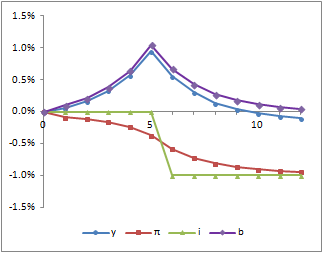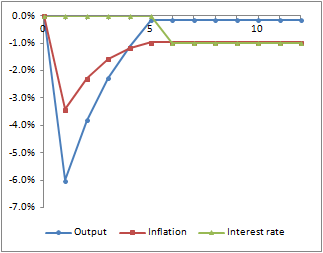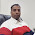Saturday, 26 December 2015

Fiscal Policy in Neo-Fisherite Versions of New Keynesian Models

This post is intended to enable me to explain in a bit more detail something I have been describing in an exchange with Stephen Williamson on his blog.

The context is how fiscal policy might impact on the results of models of interest rate changes, particularly in the impulse response on output.  I have in mind here a model based on John Cochrane's, which I've looked at before.  The two main equations are a New Keynesian IS curve and Phillips curve.  There are also assumed to be lump sum taxes and single period government bonds, so there needs to be a government budget constraint which determines the evolution of the stock of bonds.  The three equations in y (output), π (inflation) and b (the real end period value of the stock of bonds) are:

(1)          yt = E[yt+1] . ( β . it+1 / E[πt+1] )

(2)          πt = E[πt+1]β . ytκ

(3)          bt = bt-1 . it / πt - τt

where i is the interest rate and τ is the level of real lump sum taxes.

Here, monetary policy is an interest rate peg with i as the policy tool - we have no central bank reaction function.  We want to consider the impact of a 1% cut in this rate, announced five period in advance.  In the first instance, we will assumes that fiscal policy involves keeping τ constant.

The results of this are shown below (showing deviations from starting values).Inflation starts to decline immediately and eventually reflects the drop in nominal interest rates.  This means that, prior to the actual rate cut, real interest rates are higher, which implies that output rises.  Once actual nominal rates are cut, real rates are lower and output declines again.

This change results in a temporary increase in the real stock of bonds.  This happens because real rates have risen and real rates are a key factor in determining how the stock of bonds changes over time.

So I want to consider an alternative fiscal policy rule; one where the level of taxes, τt, is adjusted to prevent bt ever rising above b0.  We therefore need a fourth equation which is:

(4)          τt = GREATER OF { τ0 } OR { bt-1 . it / πt - b0 }

Adding in this equation gives us the results shown below (bt = b0 for all t, so I have not included it in the chart).Under this fiscal policy rule, both output and inflation drop sharply on the announcement, and then both rise until the actual rate change takes place.  These paths clearly satisfy equations (1) and (2) for every period where there is no surprise.  Output is rising when inflation is lower than nominal interest rates; inflation is rising when output is below its equilibrium level.  Equations (3) and (4) selects this equilibrium path from multiple others that would also satisfy (1) and (2) (although I cannot be sure that there are no other alternative paths that would satisfy all four equations).

Note on parameters.  I have used Cochrane's values of β = 0.97 and κ = 0.2, (as well as assuming a τ0 equal to 20% of y0).  These parameters suggest time periods in the order of a year, so we are talking about changes announced five years in advance.  If we adjust the parameters to reflect shorter periods (like a month), then announcing five periods in advance has a more limited impact (although still the same pattern).

1 comment:

1.Find vehicle transportation company in dilshad gargen.
Car and Bike Transport in Dilshad Garden